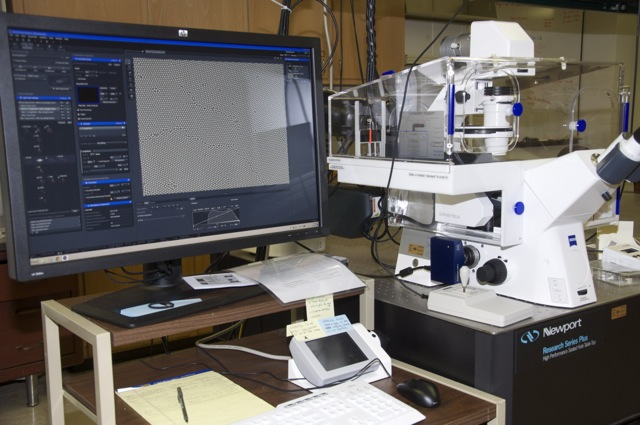Lab Report TemplateThis is a great place to start for your technical report. The templates are in both LaTeX and Word formats Laboratory formula cheat sheetPrint out this handy summary of error-propagation formulas and tape it to the inside front cover of your lab notebook Basic data analysis Why do we use the mean, standard deviation, and standard error to describe a set of measurements? Least-squares fitting How do you determine the best line (or other curve) that agrees with your data? ExcelUsing a spreadsheet to help analyze data Igor ProUsing Igor Pro to analyze data Wolfram Alpha Why think when you can ask Wolfram Alpha? Well, actually, there are many good reasons... Units calculator Do you find this units calculator convenient?